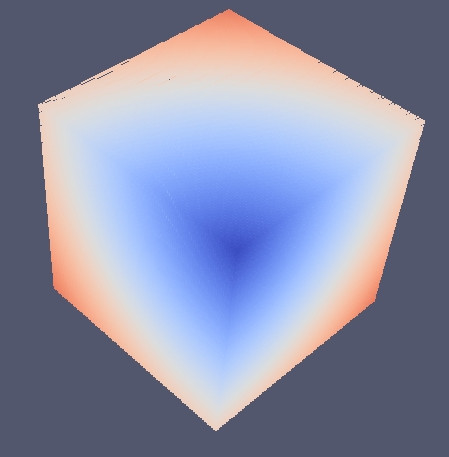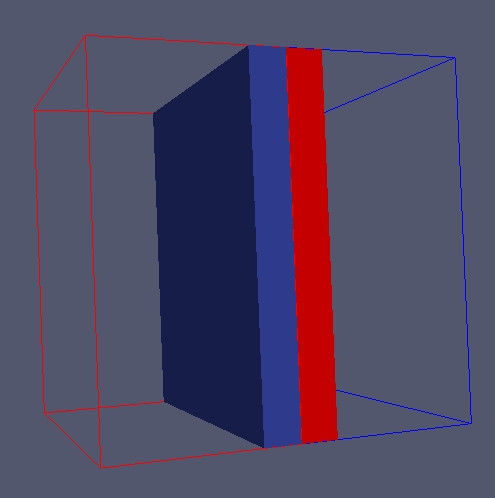#include "Grid/grid_dist_id.hpp" #include "data_type/aggregate.hpp" /*! \page grid Grid * * \subpage grid_0_simple * \subpage grid_1_save_load * \subpage Grid_1_stencil * \subpage Grid_2_solve_eq * \subpage Grid_3_gs * \subpage Grid_3_gs_3D * */ /*! \page grid_0_simple Simple usage [TOC] # Simple grid example # {#simple_grid_example} This example show several basic functionalities of the distributed grid \htmlonly Video \endhtmlonly */ int main(int argc, char* argv[]) { /*! \page grid_0_simple Simple usage * * ## Initialization ## {#e0_s_initialization} * * Here we: * * Initialize the library * * Create A 3D box that define our the domain * * an array of 3 unsigned integer that will define the size of the grid on each dimensions * * A Ghost object that will define the extension of the ghost part in physical units * * \snippet Grid/0_simple/main.cpp initialization * */ //! \cond [initialization] \endcond // Initialize the library openfpm_init(&argc,&argv); // 3D physical domain Box<3,float> domain({0.0,0.0,0.0},{1.0,1.0,1.0}); // Grid size on eaxh dimension size_t sz = {100,100,100}; // Ghost part Ghost<3,float> g(0.1); //! \cond [initialization] \endcond /*! \page grid_0_simple Simple usage * * ## Grid instantiation ## {#e0_s_grid_inst} * * Here we are creating a distributed grid defined by the following parameters * * * 3 dimensionality of the grid * * float type used for the spatial coordinates * * each grid point contain a vector of dimension 3 (float), * * float is the information stored by each grid point a float * the list of properties must be put into an aggregate data structure aggregate * * Constructor parameters: * * * sz: size of the grid on each dimension * * domain: where the grid is defined * * g: ghost extension * * \snippet Grid/0_simple/main.cpp grid instantiation * */ //! \cond [grid instantiation] \endcond grid_dist_id<3, float, aggregate> g_dist(sz,domain,g); //! \cond [grid instantiation] \endcond /*! * \page grid_0_simple Simple usage * * ## Loop over grid points ## {#e0_s_loop_gp} * * Get an iterator that go through all the grid points. In this * example we use iterators. Iterators are convenient way to explore/iterate data-structures. * * \snippet Grid/0_simple/main.cpp get iterator * \snippet Grid/0_simple/main.cpp get iterator2 * */ //! \cond [get iterator] \endcond // Get the iterator (No ghost) auto dom = g_dist.getDomainIterator(); // Counter size_t count = 0; // Iterate over all the grid points while (dom.isNext()) { //! \cond [get iterator] \endcond /*! * \page grid_0_simple Simple usage * * ## Grid coordinates ## {#e0_s_grid_coord} * * Get the local grid key, one local grid key* identify one point in the grid and store the local grid coordinates of such point * * (*)Internally a local grid store the sub-domain id (each sub-domain contain a grid) and the local grid point id identified by 2 integers in 2D 3 integer in 3D and so on. These two distinct elements are available with key.getSub() and key.getKey(). * * \snippet Grid/0_simple/main.cpp local grid * */ //! \cond [local grid] \endcond // local grid key from iterator auto key = dom.get(); //! \cond [local grid] \endcond /*! * \page grid_0_simple Simple usage * * **Short explanation** * * In oder to get the real/global coordinates of the grid point we have to convert the object key with getGKey * */ /*! * * \page grid_0_simple Simple usage * \htmlonly Long Explanation \endhtmlonly * * \htmlonly \endhtmlonly */ /*! \page grid_0_simple Simple usage * * \snippet Grid/0_simple/main.cpp global coord * */ //! \cond [global coord] \endcond auto key_g = g_dist.getGKey(key); //! \cond [global coord] \endcond /*! * \page grid_0_simple Simple usage * * ## Assign properties ## {#grid_assign} * * Each grid point has a vector property we write on the vector coordinates the global coordinate of the grid point. * At the same time we also count the points * * \snippet Grid/0_simple/main.cpp assign * */ //! \cond [assign] \endcond g_dist.template get<0>(key) = key_g.get(0); g_dist.template get<0>(key) = key_g.get(1); g_dist.template get<0>(key) = key_g.get(2); // Count the points count++; //! \cond [assign] \endcond //! \cond [get iterator2] \endcond // next point ++dom; } //! \cond [get iterator2] \endcond /*! * \page grid_0_simple Simple usage * * Each sub-domain has an extended part, that is materially contained in * another processor. The function ghost_get guarantee (after return) that this extended part * is perfectly synchronized with the other processor. * * \snippet Grid/0_simple/main.cpp ghost get * */ //! \cond [ghost get] \endcond g_dist.template ghost_get<0>(); //! \cond [ghost get] \endcond /*! * \page grid_0_simple Simple usage * * count contain the number of points the local processor contain, if we are interested to count the total number across the processor * we can use the function sum, to sum numbers across processors. First we have to get an instance of Vcluster, queue an operation of sum with * the variable count and finally execute. All the operation are asynchronous, execute work like a barrier and ensure that all the * queued operations are executed * * \snippet Grid/0_simple/main.cpp reduce * */ //! \cond [reduce] \endcond // Get the VCluster object Vcluster & vcl = create_vcluster(); // queue an operation of sum for the counter count vcl.sum(count); // execute the operation vcl.execute(); // only master output if (vcl.getProcessUnitID() == 0) std::cout << "Number of points: " << count << "\n"; //! \cond [reduce] \endcond /*! * \page grid_0_simple Simple usage * * ## VTK and visualization ## {#e0_s_VTK_vis} * * Finally we want a nice output to visualize the information stored by the distributed grid. * The function write by default produce VTK files. One for each processor that can be visualized * with the programs like paraview * * \htmlonly ** \endhtmlonly * * \snippet Grid/0_simple/main.cpp write * */ //! \cond [write] \endcond g_dist.write("output"); //! \cond [write] \endcond /*! * \page grid_0_simple Simple usage * * ## Decomposition ## {#grid_dec} * * For debugging purpose and demonstration we also output the decomposition of the * space across processor. This function produce VTK files that can be visualized with Paraview * * \snippet Grid/0_simple/main.cpp out_dec * * \htmlonly ** \endhtmlonly * * Here we see the decomposition in 3D for 2 processors. The red box in wire-frame is the processor 0 * subdomain. The blu one is the processor 1 sub-domain. The red solid box is the extended part for processor 0 * the blu solid part is the extended part for processor 1 * * */ //! \cond [out_dec] \endcond g_dist.getDecomposition().write("out_dec"); //! \cond [out_dec] \endcond /*! * \page grid_0_simple Simple usage * * ## Finalize ## {#finalize} * * At the very end of the program we have always to de-initialize the library * * \snippet Vector/0_simple/main.cpp finalize * */ //! \cond [finalize] \endcond openfpm_finalize(); //! \cond [finalize] \endcond /*! * \page grid_0_simple Simple usage * * # Full code # {#code} * * \include Grid/0_simple/main.cpp * */ }